Smartick is an advanced online program that teaches kids math and coding in only 15 min. a day

Jan26

# Inverse Proportionality: The Rule of Three Inverse

In this week’s post, we’re going to look at what the rule of three inverse is and how to use it to solve problems.

The rule of three is a way to solve proportionality problems. If we are dealing with a direct proportionality, then apply the direct rule of three. If we are dealing with an inverse proportionality, we apply the rule of three inverse.

Let’s take a look at what inverse proportionality is so that we can see learn how to apply it later on in this post.

### Inverse Proportionality

We have 2 magnitudes (A and B) and we need to observe the relationship that exists between the two:

As A gets bigger, B gets smaller. This means that the proportion between the two magnitudes is inverse.

For example, in the following table, you can see A and B’s magnitudes: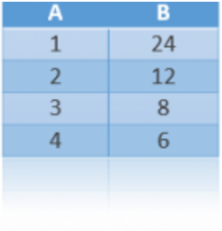It’s an inverse proportionality because as long as A gets bigger, B gets smaller.

### How do we use the rule of three inverse?

The rule of three inverse is used in problems where three of the four numbers that are proportional are known and the fourth number is what needs to be solved.

First, we need to relate A to B. Then, we write down the next pair of numbers that we need to relate.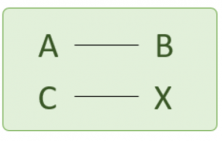C is magnitude A’s value and X, the value we are solving for, is magnitude B’s value.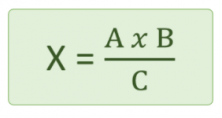### How do we solve a problem with the rule of three inverse?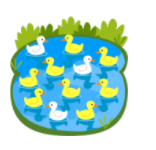At a farm, it takes 10 days for 20 ducks to eat the food that’s left out for them. How much time would it take for 40 ducks to eat the same food?

First, we have to determine if the proportionality is direct or inverse:

It takes 10 days for 20 ducks. 40 ducks…would it take them more or less time to eat the food?

More ducks means that the food will run out faster, so, it’ll take them less time to eat the food.

If the number of ducks increases, the number of days decreases. We are looking at an inverse proportionality.

Now, let’s apply the rule of 3 inverse: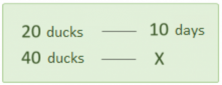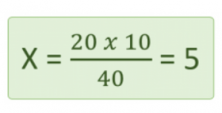It will take 5 days for 40 ducks to eat all the food.It takes 12 days for 3 painters to paint a house. How long will it take for 9 painters to do the same job?

First, we need to determine which kind of proportionality it is.

It takes 12 days for 3 painters. 9 painters…will they take more or less time to do the job?

More painters means that it’ll take less time for them to finish the job. So, we’re dealing with an inverse proportionality.

Now, we just need to apply the rule of three inverse: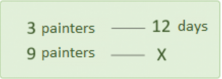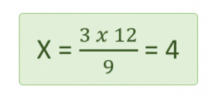It takes 4 days for 9 painters to paint the house.

If you want to keep learning and practicing Elementary math, make an account with Smartick.

Latest posts by Smartick (see all)